## Compound Interest Formula

Whenever we borrow money from someone, an amount of interest gets added along with. That amount of interest is Compound Interest and here we are providing you Compound Interest Formula details. All the details that are concerned with the Compound Interest like use of Compound Interest Formula In Excel , Calculator, Example & Derivation of Compound Interest etc. compound Interest in calculated on various basis like monthly basis, quarterly basis (after every four month), half yearly basis (after every 6 months) and annually (once in a year). With the help of the Formula of Compound Interest it will become easier to calculate the amount of interest to be paid and to be received. To know more lets take a glance over this whole page…

• Formulas Of Compound Interest With Example
• Compound Interest Formula Excel
• Compound Interest Formulas PDF
• How to calculate Monthly/ Quarterly/ Half Yearly/ Annually Compound Interest
• Compound Interest Solved Question and Answer
The whole usage of Compound Interest Formula in Maths is described here on this page. via the details given you can get to know about Compound Interest Formula Calculator India and you will also get details about the usage of Compound Interest in real life. Compound interest formulas are also asked in examination of 9th, 10th standard and sometimes in the competitive examinations too. Keeping all these facts in mind we the team members of www.privatejobshub.in have given the details about the usage of Compound Interest Formula In Excel and all other relevant facts.

### Compound Interest FormulaCompound Interest Formula In Excel

1. Suppose you put Rs.100 into a bank. How much will your investment be worth after one year at an annual interest rate of 8%? The answer is Rs.108.2. Now this interest (Rs.8) will also earn interest (compound interest) next year. How much will your investment be worth after two years at an annual interest rate of 8%? The answer is Rs.116.64.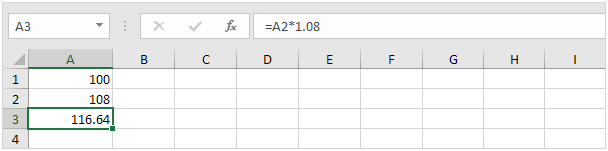3. How much will your investment be worth after 5 years? Simply drag the formula down to cell A6.4. All we did was multiplying 100 by 1.08, 5 times. So we can also directly calculate the value of the investment after 5 years.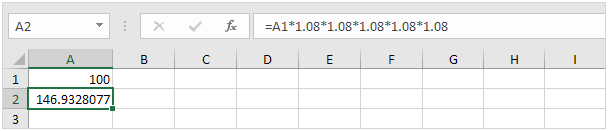which is the same as:Note: There is no special function for compound interest in Excel. However, you can easily create a compound interest calculator to compare different rates and different durations.

5. Assume you put Rs.10,000 into a bank. How much will your investment be worth after 10 years at an annual interest rate of 5% compounded monthly? The answer is Rs.16,470.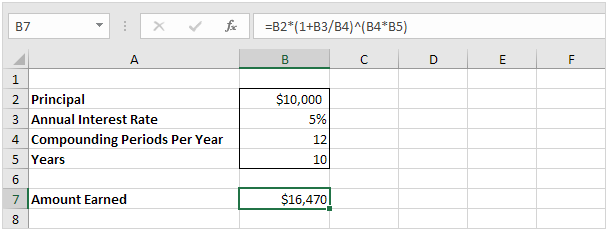6. Assume you put Rs.10,000 into a bank. How much will your investment be worth after 15 years at an annual interest rate of 4% compounded quarterly? The answer is Rs.18,167.Compound Interest Formula Calculation

How to calculate Monthly/ Quarterly/ Half Yearly/ Annually Compound Interest with Example?
Here in the below section we are going to provide you the Compound Interest Formula With Example. Here we have stated Compound Interest Formula For Half Yearly, Compound Interest Formula For Quarterly, Compound Interest Formula For Monthly and Compound Interest Formula For Annually and also have given some questions which will clear your visions more.

Compound Interest Formula Example

Monthly Calculation of Compound Interest:

1. Find the compound interest on Rs. 2,000 at 4% per Month for 1 year, compounded monthly?
Formula Used: Compound Interest Formula Monthly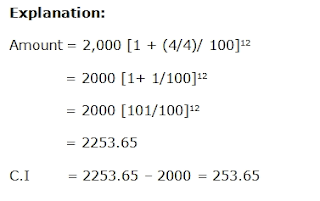Quarterly Calculation of Compound Interest:

1. Find the compound interest on Rs.16,000 at 20% per annum for 9 months, compounded quarterly
Formula Used:Compound Interest Formula Quarterly

Explanation:Half Yearly Calculation of Compound Interest:

1. A bank offers 5% compound interest calculated on half-yearly basis. A customer deposits Rs. 1600 each on 1st January and 1st July of a year. At the end of the year, the amount he would have gained by way of interest is:
Formula Used:Compound Interest Formula Half-YearlyAnnually Calculation of Compound Interest:

1. Find the compound interest on Rs. 7500 at 4% per annum for 2 years, compounded annually.
Formula Used:Compound Interest Formula Annually

Explanation: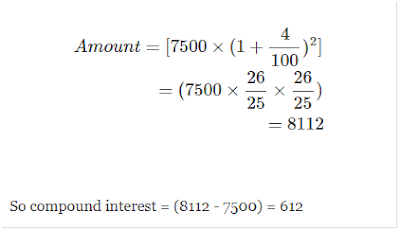1. There is 60% increase in an amount in 6 years at simple interest. What will be the compound interest of Rs. 12,000 after 3 years at the same rate?
1. Rs. 2160
2. Rs. 3120
3. Rs. 3972
4. Rs. 6240
5. None of these

Explanation: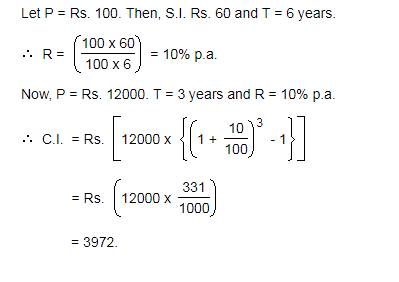2.            The compound interest on Rs. 30,000 at 7% per annum is Rs. 4347. The period (in years) is:

1. 2
2. 5/2
3. 3
4. 4

Explanation: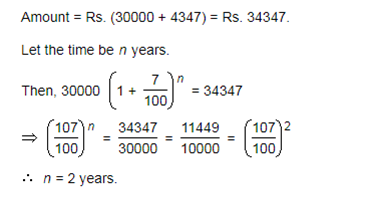3.            What will be the compound interest on a sum of Rs. 25,000 after 3 years at the rate of 12 p.c.p.a.?

1. Rs. 9000.30
2. Rs. 9720
3. Rs. 10123.20
4. Rs. 10483.20
5. None of these

Explanation:4.            The difference between simple and compound interests compounded annually on a certain sum of money for 2 years at 4% per annum is Re. 1. The sum (in Rs.) is:

1. 625
2. 630
3. 640
4. 650

Want help in Preparation? Get Tips: Practice Which Helps In Preparation

Explanation:5.            What is the difference between the compound interests on Rs. 5000 for 1 years at 4% per annum compounded yearly and half-yearly?

1. Rs. 2.04
2. Rs. 3.06
3. Rs. 4.80
4. Rs. 8.30

 Take a Test  Now

Explanation: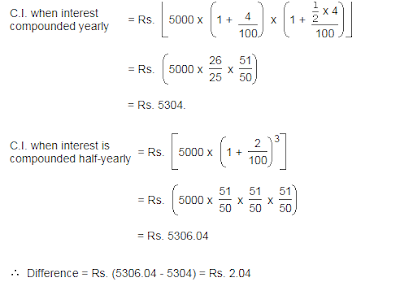6.            At what rate of compound interest per annum will a sum of Rs. 1200 become Rs. 1348.32 in 2 years?

1. 6%
2. 6.5%
3. 7%
4. 7.5%

7.            Albert invested an amount of Rs. 8000 in a fixed deposit scheme for 2 years at compound interest rate 5 p.c.p.a. How much amount will Albert get on maturity of the fixed deposit?

1. Rs. 8600
2. Rs. 8620
3. Rs. 8820
4. None of these

8.            The effective annual rate of interest corresponding to a nominal rate of 6% per annum payable half-yearly is:

1. 6.06%
2. 6.07%
3. 6.08%
4. 6.09%
9.            The least number of complete years in which a sum of money put out at 20% compound interest will be more than doubled is:

1. 3
2. 4
3. 5
4. 6

10.         Simple interest on a certain sum of money for 3 years at 8% per annum is half the compound interest on Rs. 4000 for 2 years at 10% per annum. The sum placed on simple interest is:

1. Rs. 1550
2. Rs. 1650
3. Rs. 1750
4. Rs. 2000

11.         The difference between simple interest and compound on Rs. 1200 for one year at 10% per annum reckoned half-yearly is:

1. Rs. 2.50
2. Rs. 3
3. Rs. 3.75
4. Rs. 4
5. None of these

12.         The compound interest on a certain sum for 2 years at 10% per annum is Rs. 525. The simple interest on the same sum for double the time at half the rate percent per annum is:

1. Rs. 400
2. Rs. 500
3. Rs. 600
4. Rs. 800

13.         The difference between compound interest and simple interest on an amount of Rs. 15,000 for 2 years is Rs. 96. What is the rate of interest per annum?

1. 8
2. 10
3. 12
4. Cannot be determined
5. None of these

Look On: Bank Syllabus

14.         If the simple interest on a sum of money for 2 years at 5% per annum is Rs. 50, what is the compound interest on the same at the same rate and for the same time?

1. Rs. 51.25
2. Rs. 52
3. Rs. 54.25
4. Rs. 60
15.         The difference between the compound interest compounded annually and simple interest for 2 years at 20% per annum is Rs.144. Find the principal?

1. Rs. 3000
2. Rs. 3300
3. Rs. 3600
4. Rs. 3900

Press To See: Bank Previous Papers

Check On: Bank Exam Pattern

Compound Interest Formula

Here in the above section of this page we have provided all the details regarding the Compound Interest Formula India. In our country most of the savings are deposited in banks and on the basis of the amount we deposit the banks gives us a particular amount of interested. As same as banks if we borrow money from a third part (other than bank) than also we pay interest on the amount we borrow. The formulas given above are used to calculate that interested which is also called as Compound Interest. There are many online applications available through which you can check the Compound interest Compound Interest Formula Online and also get Compound Interest Formula Aptitude. Compound interest Depends on money deposited (principal), the annual interest rate, number of times the money is compounded per year and years for which money is left in the bank.